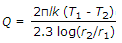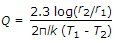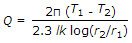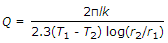# Mechanical Engineering - Heat Transfer, Refrigeration and Air Conditioning

### Exercise :: Heat Transfer, Refrigeration and Air Conditioning - Section 4

36.

A refrigerating system operating on reversed Brayton refrigeration cycle is used for maintaining 250 K. If the temperature at the end of constant pressure cooling is 300 K and rise in the temperature of air in the refrigerator is 50 K, then the net work of compression will be (assume air as working substance with cp = 1 kJ/kg)

 A. 25 kJ/kg B. 50 kJ/kg C. l00 kJ/kg D. 125 kJ/kg

Explanation:

No answer description available for this question. Let us discuss.

37.

Wet bulb temperature indicates the moisture content in air.

 A. Agree B. Disagree

Explanation:

No answer description available for this question. Let us discuss.

38.

A designer chooses the values of fluid flow rates and specific heats in such a manner that the heat capacities of the two fluids are equal. A hot fluid enters the counter flow heat exchanger at 100° C and leaves at 60° C. A cold fluid enters the heat exchanger at 40° C. The mean temperature difference between the two fluids is

 A. 20°C B. 40°C C. 60°C D. 66.7°C

Explanation:

No answer description available for this question. Let us discuss.

39.

The inclined and non-uniformly spaced straight lines on a psychrometric chart indicates wet bulb temperature.

 A. Agree B. Disagree

Explanation:

No answer description available for this question. Let us discuss.

40.

The heat transfer by conduction through a thick cylinder(Q) is given by (where T1 = Higher temperature, T2 = Lower temperature, r1 = Inside radius, r2 = Outside radius, l = Length of cylinder, and k = Thermal conductivity)

 A.B.C.D.